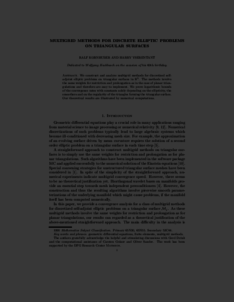Repository: Freie Universität Berlin, Math Department

# Multigrid methods for discrete elliptic problems on triangular surfaces

Kornhuber, R. and Yserentant, H. (2008) Multigrid methods for discrete elliptic problems on triangular surfaces. Computing and Visualization in Science, 11 (4-6). pp. 251-257. ISSN 1432-9360Preview

168kB

Official URL: http://dx.doi.org/10.1007/s00791-008-0102-4

## Abstract

We construct and analyze multigrid methods for discretized self-adjoint elliptic problems on triangular surfaces in R3. The methods involve the same weights for restriction and prolongation as in the case of planar triangulations and therefore are easy to implement. We prove logarithmic bounds of the convergence rates with constants solely depending on the ellipticity, the smoothers and on the regularity of the triangles forming the triangular surface. Our theoretical results are illustrated by numerical computations.

Item Type: Article Mathematical and Computer Sciences > Mathematics > Numerical Analysis Department of Mathematics and Computer Science > Institute of Mathematics 1808 Ekaterina Engel 19 Feb 2016 08:50 03 Mar 2017 14:41

Repository Staff Only: item control page